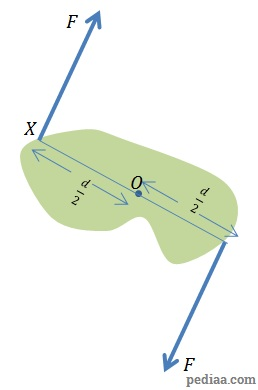# Difference Between Moment and Couple

## Main Difference – Moment vs. Couple

In physics, the terms moment and couple are related to forces that cause turning effects on bodies. The main difference between moment and couple is that, in a moment, there may or may not be a resultant force as a consequence of the forces causing the turning effect whereas, in a couple, there is no resultant force.

## What is Moment

Technically, a moment is a combination of a physical quantity and a distance. However, in this article we use the term “moment” to mean the “torque” that an object experiences due to a force. The torque$\vec{\tau}$ about any point due to a force$\vec{F}$ is given by the cross product between$\vec{F}$ and the vector$\vec{r}$ drawn from the point we are interested in, to the point where force is applied:$\vec{\tau}=\vec{r}\times \vec{F}$Defining Torque

The magnitude of torque could be given by the product of force and perpendicular distance to the line of action of force:Finding the magnitude of torque using perpendicular distance to the line of action of force.

So in this case,$\left | \vec{\tau} \right |=\left | \vec{F} \right |d$

## What is Couple

A couple describes a system where there is a resultant torque by a system of forces, without there being a resultant force. Let us look at a system where a pair of forces, each with a magnitude$F$, separated by a perpendicular distance$d$ form a couple.

Since the net force must be 0, it follows that the forces must be acting in opposite directions and also that they have the same magnitudes. We calculate the torque due to these forces below.A couple

Let us consider the torque at$O$. This is the midpoint between the two forces. Each force contributes to a clockwise turning effect about this point. So, the total (clockwise) moment about O is:$\tau=F\left( \frac{d}{2}\right)+F\left( \frac{d}{2}\right)=Fd$

Note that for a couple, the resultant torque is always the same regardless of about which point the moments are taken. In the above example, we can confirm this by taking the moment about point$X$. In this case, the torque by the force at$X$ is 0 (because the distance to the force is 0). Then, the net torque is only due to the force at the other end of the object. This force is at a perpendicular distance$d$ away, so the torque is:$\tau =Fd$

Examples of couples involve forces applied by hands on a steering wheel, forces on the head of a screwdriver and forces acting on pedals of a bicycle.The force exerted by hands on a steering wheel form a couple

## Difference Between Moment and Couple

### Resultant Force:

A moment may or may not accompany a resultant force.

A couple does not accompany a resultant force.

### Effect of Force:

A moment can occur with only one force.

But, a couple cannot be produced by a single force.

Image Courtesy:

“Hands on a steering wheel.” by Vernon Chan [CC BY 2.0], via Wikimedia Commons